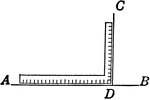### Right Angle

Right angle with square at perpendicular lines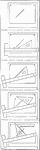### Drawing Perpendicular Lines Exercise

Lines perpendicular to each other may be drawn by using a triangle in combination with the T-square.…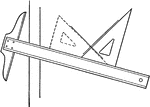### Drawing Perpendicular Lines Using T-square and Triangle

Perpendicular lines are drawn by fitting the hypothenuse of the triangle to the T-square at an angle,…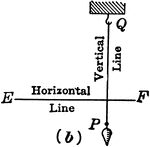### Perpendicular Lines

Right angle at perpendicular lines demonstrated by string on plumb line.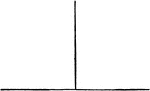### Perpendicular Lines

Illustration of perpendicular lines. Intersecting lines that meet at a right angle.### Perpendicular Lines With Horizontal and Vertical Labeled

Illustration of perpendicular lines with the horizontal and vertical lines labeled. "A horizontal line…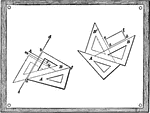### Mechanical Drawing Exercise

Drawing perpendicular lines using a drawing board and pairs of triangles.